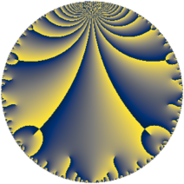# Properties

 Label 722.6.aLevel $722$ Weight $6$ Character orbit 722.a Rep. character $\chi_{722}(1,\cdot)$ Character field $\Q$ Dimension $143$ Newform subspaces $20$ Sturm bound $570$ Trace bound $3$

# Related objects

## Defining parameters

 Level: $$N$$ $$=$$ $$722 = 2 \cdot 19^{2}$$ Weight: $$k$$ $$=$$ $$6$$ Character orbit: $$[\chi]$$ $$=$$ 722.a (trivial) Character field: $$\Q$$ Newform subspaces: $$20$$ Sturm bound: $$570$$ Trace bound: $$3$$ Distinguishing $$T_p$$: $$3$$

## Dimensions

The following table gives the dimensions of various subspaces of $$M_{6}(\Gamma_0(722))$$.

Total New Old
Modular forms 495 143 352
Cusp forms 455 143 312
Eisenstein series 40 0 40

The following table gives the dimensions of the cuspidal new subspaces with specified eigenvalues for the Atkin-Lehner operators and the Fricke involution.

$$2$$$$19$$FrickeDim.
$$+$$$$+$$$$+$$$$35$$
$$+$$$$-$$$$-$$$$37$$
$$-$$$$+$$$$-$$$$39$$
$$-$$$$-$$$$+$$$$32$$
Plus space$$+$$$$67$$
Minus space$$-$$$$76$$

## Trace form

 $$143q - 4q^{2} + 4q^{3} + 2288q^{4} - 44q^{5} - 8q^{6} - 76q^{7} - 64q^{8} + 11929q^{9} + O(q^{10})$$ $$143q - 4q^{2} + 4q^{3} + 2288q^{4} - 44q^{5} - 8q^{6} - 76q^{7} - 64q^{8} + 11929q^{9} - 200q^{10} - 162q^{11} + 64q^{12} - 1338q^{13} - 80q^{14} - 1868q^{15} + 36608q^{16} + 934q^{17} - 628q^{18} - 704q^{20} + 2940q^{21} + 1424q^{22} + 4068q^{23} - 128q^{24} + 97427q^{25} + 2144q^{26} - 3464q^{27} - 1216q^{28} + 5566q^{29} + 7792q^{30} + 11164q^{31} - 1024q^{32} + 6800q^{33} - 3080q^{34} + 4264q^{35} + 190864q^{36} - 10038q^{37} - 11460q^{39} - 3200q^{40} - 25722q^{41} - 8656q^{42} + 998q^{43} - 2592q^{44} - 19720q^{45} - 688q^{46} - 2400q^{47} + 1024q^{48} + 369203q^{49} + 16260q^{50} - 47816q^{51} - 21408q^{52} - 53370q^{53} + 42208q^{54} - 33836q^{55} - 1280q^{56} + 14640q^{58} + 31028q^{59} - 29888q^{60} - 133476q^{61} + 46192q^{62} + 54796q^{63} + 585728q^{64} + 108904q^{65} + 62304q^{66} + 29516q^{67} + 14944q^{68} + 121196q^{69} - 65232q^{70} - 5892q^{71} - 10048q^{72} + 199254q^{73} + 14432q^{74} + 136620q^{75} - 47832q^{77} + 2800q^{78} - 202780q^{79} - 11264q^{80} + 1283759q^{81} + 71272q^{82} - 134810q^{83} + 47040q^{84} + 176372q^{85} + 83968q^{86} + 232284q^{87} + 22784q^{88} - 202750q^{89} - 85720q^{90} - 481736q^{91} + 65088q^{92} - 225568q^{93} - 61312q^{94} - 2048q^{96} - 16390q^{97} - 243076q^{98} - 260246q^{99} + O(q^{100})$$

## Decomposition of $$S_{6}^{\mathrm{new}}(\Gamma_0(722))$$ into newform subspaces

Label Dim. $$A$$ Field CM Traces A-L signs $q$-expansion
$$a_2$$ $$a_3$$ $$a_5$$ $$a_7$$ 2 19
722.6.a.a $$1$$ $$115.797$$ $$\Q$$ None $$-4$$ $$14$$ $$-45$$ $$-121$$ $$+$$ $$-$$ $$q-4q^{2}+14q^{3}+2^{4}q^{4}-45q^{5}-56q^{6}+\cdots$$
722.6.a.b $$1$$ $$115.797$$ $$\Q$$ None $$4$$ $$6$$ $$31$$ $$-27$$ $$-$$ $$-$$ $$q+4q^{2}+6q^{3}+2^{4}q^{4}+31q^{5}+24q^{6}+\cdots$$
722.6.a.c $$2$$ $$115.797$$ $$\Q(\sqrt{1441})$$ None $$8$$ $$-3$$ $$-45$$ $$114$$ $$-$$ $$-$$ $$q+4q^{2}+(-1-\beta )q^{3}+2^{4}q^{4}+(-24+\cdots)q^{5}+\cdots$$
722.6.a.d $$3$$ $$115.797$$ $$\mathbb{Q}[x]/(x^{3} - \cdots)$$ None $$-12$$ $$-13$$ $$81$$ $$228$$ $$+$$ $$-$$ $$q-4q^{2}+(-4-\beta _{1})q^{3}+2^{4}q^{4}+(3^{3}+\cdots)q^{5}+\cdots$$
722.6.a.e $$3$$ $$115.797$$ $$\mathbb{Q}[x]/(x^{3} - \cdots)$$ None $$-12$$ $$15$$ $$-14$$ $$-312$$ $$+$$ $$+$$ $$q-4q^{2}+(5-\beta _{1})q^{3}+2^{4}q^{4}+(-5+\cdots)q^{5}+\cdots$$
722.6.a.f $$3$$ $$115.797$$ $$\mathbb{Q}[x]/(x^{3} - \cdots)$$ None $$12$$ $$-15$$ $$-14$$ $$-312$$ $$-$$ $$-$$ $$q+4q^{2}+(-5+\beta _{1})q^{3}+2^{4}q^{4}+(-5+\cdots)q^{5}+\cdots$$
722.6.a.g $$4$$ $$115.797$$ $$\mathbb{Q}[x]/(x^{4} - \cdots)$$ None $$-16$$ $$-14$$ $$36$$ $$38$$ $$+$$ $$-$$ $$q-4q^{2}+(-3-\beta _{1})q^{3}+2^{4}q^{4}+(9+\cdots)q^{5}+\cdots$$
722.6.a.h $$4$$ $$115.797$$ $$\mathbb{Q}[x]/(x^{4} - \cdots)$$ None $$-16$$ $$1$$ $$-14$$ $$97$$ $$+$$ $$+$$ $$q-4q^{2}+\beta _{1}q^{3}+2^{4}q^{4}+(-4+\beta _{1}+\cdots)q^{5}+\cdots$$
722.6.a.i $$4$$ $$115.797$$ $$\mathbb{Q}[x]/(x^{4} - \cdots)$$ None $$16$$ $$-1$$ $$-14$$ $$97$$ $$-$$ $$+$$ $$q+4q^{2}-\beta _{1}q^{3}+2^{4}q^{4}+(-4+\beta _{1}+\cdots)q^{5}+\cdots$$
722.6.a.j $$4$$ $$115.797$$ $$\mathbb{Q}[x]/(x^{4} - \cdots)$$ None $$16$$ $$14$$ $$36$$ $$38$$ $$-$$ $$+$$ $$q+4q^{2}+(3+\beta _{1})q^{3}+2^{4}q^{4}+(9+\beta _{2}+\cdots)q^{5}+\cdots$$
722.6.a.k $$6$$ $$115.797$$ $$\mathbb{Q}[x]/(x^{6} - \cdots)$$ None $$-24$$ $$24$$ $$-83$$ $$104$$ $$+$$ $$-$$ $$q-4q^{2}+(5-\beta _{1}-\beta _{2})q^{3}+2^{4}q^{4}+\cdots$$
722.6.a.l $$6$$ $$115.797$$ $$\mathbb{Q}[x]/(x^{6} - \cdots)$$ None $$24$$ $$-24$$ $$-83$$ $$104$$ $$-$$ $$-$$ $$q+4q^{2}+(-5+\beta _{1}+\beta _{2})q^{3}+2^{4}q^{4}+\cdots$$
722.6.a.m $$8$$ $$115.797$$ $$\mathbb{Q}[x]/(x^{8} - \cdots)$$ None $$-32$$ $$22$$ $$-108$$ $$94$$ $$+$$ $$-$$ $$q-4q^{2}+(3-\beta _{1})q^{3}+2^{4}q^{4}+(-13+\cdots)q^{5}+\cdots$$
722.6.a.n $$8$$ $$115.797$$ $$\mathbb{Q}[x]/(x^{8} - \cdots)$$ None $$32$$ $$-22$$ $$-108$$ $$94$$ $$-$$ $$-$$ $$q+4q^{2}+(-3+\beta _{1})q^{3}+2^{4}q^{4}+(-13+\cdots)q^{5}+\cdots$$
722.6.a.o $$12$$ $$115.797$$ $$\mathbb{Q}[x]/(x^{12} - \cdots)$$ None $$-48$$ $$0$$ $$-42$$ $$-438$$ $$+$$ $$+$$ $$q-4q^{2}+\beta _{1}q^{3}+2^{4}q^{4}+(-3+\beta _{7}+\cdots)q^{5}+\cdots$$
722.6.a.p $$12$$ $$115.797$$ $$\mathbb{Q}[x]/(x^{12} - \cdots)$$ None $$48$$ $$0$$ $$-42$$ $$-438$$ $$-$$ $$-$$ $$q+4q^{2}-\beta _{1}q^{3}+2^{4}q^{4}+(-3+\beta _{7}+\cdots)q^{5}+\cdots$$
722.6.a.q $$15$$ $$115.797$$ $$\mathbb{Q}[x]/(x^{15} - \cdots)$$ None $$-60$$ $$0$$ $$108$$ $$84$$ $$+$$ $$-$$ $$q-4q^{2}+\beta _{1}q^{3}+2^{4}q^{4}+(7+\beta _{5})q^{5}+\cdots$$
722.6.a.r $$15$$ $$115.797$$ $$\mathbb{Q}[x]/(x^{15} - \cdots)$$ None $$60$$ $$0$$ $$108$$ $$84$$ $$-$$ $$+$$ $$q+4q^{2}-\beta _{1}q^{3}+2^{4}q^{4}+(7+\beta _{5})q^{5}+\cdots$$
722.6.a.s $$16$$ $$115.797$$ $$\mathbb{Q}[x]/(x^{16} - \cdots)$$ None $$-64$$ $$-46$$ $$84$$ $$198$$ $$+$$ $$+$$ $$q-4q^{2}+(-3+\beta _{1})q^{3}+2^{4}q^{4}+(5+\cdots)q^{5}+\cdots$$
722.6.a.t $$16$$ $$115.797$$ $$\mathbb{Q}[x]/(x^{16} - \cdots)$$ None $$64$$ $$46$$ $$84$$ $$198$$ $$-$$ $$+$$ $$q+4q^{2}+(3-\beta _{1})q^{3}+2^{4}q^{4}+(5-\beta _{1}+\cdots)q^{5}+\cdots$$

## Decomposition of $$S_{6}^{\mathrm{old}}(\Gamma_0(722))$$ into lower level spaces

$$S_{6}^{\mathrm{old}}(\Gamma_0(722)) \cong$$ $$S_{6}^{\mathrm{new}}(\Gamma_0(19))$$$$^{\oplus 4}$$$$\oplus$$$$S_{6}^{\mathrm{new}}(\Gamma_0(38))$$$$^{\oplus 2}$$$$\oplus$$$$S_{6}^{\mathrm{new}}(\Gamma_0(361))$$$$^{\oplus 2}$$